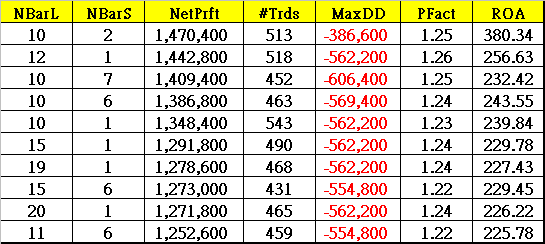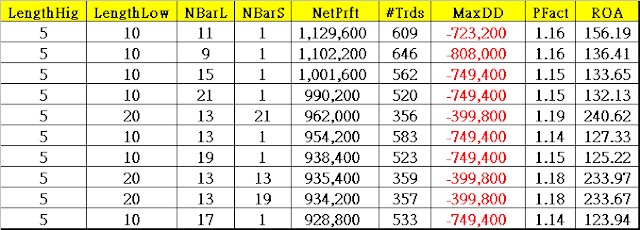## 2014年5月26日 星期一

### 順勢與逆勢策略的測試 [程式碼]

1.順勢策略 (固定及對稱的時間通道 EntryLen [M] 與多空一樣持倉時間 ExitLen [N])2.逆勢策略 (固定及對稱的時間通道 EntryLen [M] 與多空一樣的持倉時間 ExitLen [N])3.順勢策略 (固定及對稱的時間通道 EntryLen [M] 與不固定持倉時間 ExitLen [N])4.逆勢策略 (固定及對稱的時間通道 EntryLen [M] 與不固定的持倉時間 ExitLen [N])5.順勢策略 (非固定及非對稱的時間通道 EntryLen [M] 與不固定持倉時間 ExitLen [N])6.逆勢策略 (非固定及非對稱的時間通道 EntryLen [M] 與不固定的持倉時間 ExitLen [N])• 1.逆勢策略的持倉時間確實是比順勢策略來的短
• 2.非對稱式的交易邏輯績效表現比對稱式的交易邏輯好
• 3.創新高或創新低時有較大的機會回檔/反彈作逆勢交易

vars: IsBalanceDay(False),MP(0),PF(0),PL(0),DayHigh(0),DayLow(0),NBAREL(0),NBarES(0),NBarXL(0),NBarXS(0);

MP = MarketPosition ;

if DAYofMonth(Date) > 14 and DAYofMonth(Date) < 22 and DAYofWeek(Date)= 3 then isBalanceDay = True else isBalanceDay =False ;

{ for symmetrical fix BarLen }

if Symmetric = 1 then Begin { 對稱式 }
if Date <> Date then Begin {交易日替換時先計算過去高/低點 }
if DataCompression < 2 then Begin { 分 K週期 }
DayHigh = HighD(0) ;
DayLow = LowD(0) ;
For value1 = 0 to EntryLen-1 Begin
if HighD(Value1) > DayHigh then DayHigh = HighD(Value1) ;
if LowD(Value1) < DayLow then DayLow = LowD(Value1) ;
end ;
end else begin { 日K }
DayHigh = High ;
DayLow = Low ;
For value2 = 0 to EntryLen-1 Begin
if High[Value2] > DayHigh then DayHigh = High[Value2] ;
if Low[Value2] < DayLow then DayLow = Low[Value2] ;
end ;
end;
end;
end;

{ for unsymmetrical lookback Length }
if Symmetric = 2 then Begin { 非對稱時機 }
if Date <> Date then Begin {交易日替換時先計算過去高/低點 }
if DataCompression < 2 then Begin { 分 K週期 }
DayHigh = HighD(0) ;
DayLow = LowD(0) ;
For value1 = 0 to LengthHigh-1 Begin
if HighD(Value1) > DayHigh then DayHigh = HighD(Value1) ;
end ;
For value2 = 0 to LengthLow-1 Begin
if LowD(Value2) < DayLow then DayLow = LowD(Value2) ;
end ;
end else begin { 日K }
DayHigh = High ;
DayLow = Low ;
For value1 = 0 to LengthHigh-1 Begin
if High[Value1] > DayHigh then DayHigh = High[Value1] ;
end ;
For value2 = 0 to LengthLow-1 Begin
if Low[Value2] < DayLow then DayLow = Low[Value2] ;
end ;
end;
end;
end;

{ daily K }
if EntryType = 1 then Begin { 順勢 }
if MP = 0 and High = DayHigh then Buy this bar on Close ;
if MP = 0 and Low = DayLow then Sell this bar on Close ;
end;

if EntryType = 2 then Begin { 逆勢}
if MP = 0 and High = DayHigh then Sell this bar on Close ;
if MP = 0 and Low = DayLow then Buy this bar on Close ;
end;

{ 不同出場方式 }

if ExitType = 1 then SetStopLoss(PL * BigPointValue) ;

if ExitType = 2 then Begin
SetStopLoss(PL * BigPointValue) ;
SetProfitTarget(PF * BigPointValue) ;
end;

if ExitType = 3 then Begin { 固定持倉時間 }
if MP > 0 and BarsSinceEntry = ExitLen then ExitLong this bar on close ;
if MP < 0 and BarsSinceEntry = ExitLen then ExitShort this bar on close ;
end;

if ExitType = 4 then Begin { 不固定持倉時間 }
if MP > 0 and BarsSinceEntry = NBarL then ExitLong this bar on close ;
if MP < 0 and BarsSinceEntry = NBarS then ExitShort this bar on close ;
end;

if ExitType = 5 then Begin
SetStopLoss(PL * BigPointValue) ;
SetProfitTarget(PF * BigPointValue) ;
if MP > 0 and BarsSinceEntry = NBarL then Sell {ExitLong} this bar on close ;
if MP < 0 and BarsSinceEntry = NBarS then Buy {ExitShort} this bar on close ;
end;

if IsBalanceDay then setExitonClose ;